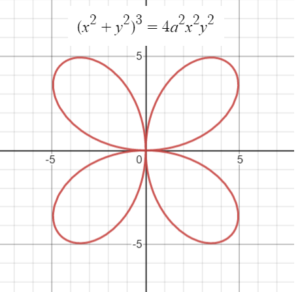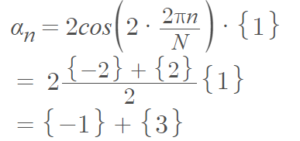The Quadrifolium (also called the Rosace a quatre jeulles or four-leaved clover) is a four-petaled rose curve with n = 2.

The quadrifolium has polar equation 

(x2 + y2)3 = 4a2x2y2

corresponding algebraic equation

(x2 + y2)3 = (x2y2)2

r = a sin(2θ).

Like all curves defined by polar coordinates as a function of trigonometric functions r = f(θ), there is an equivalent harmonic pattern function αn = f(2πn/N) · {1} with θ → 2πn/N. For a quadrifolium with equation r = 2 cos(2θ), the harmonic pattern function isThe area inside the curve is ½πa2, which corresponds to half the area of the quadrifolium’s circumcircle (or the areas complement within the curve’s circumcircle). The area is derived from integration:The arc length is s = 8 a E(½√3) = 9.6884…a
Where E(k) is a complete elliptic integral of the second kind .

## Real Life Applications of the QuadrifoliumThe curve is frequently used in modeling applications. For example, one interesting application of the quadrifolium is in simulations of hurricane flowfields. One hurricane data collection method involves flying in a figure-four pattern (i.e., a quadrifolium) through the center of a hurricane . Quadrifoliums have been used in the estimation of flowfields in general, although they made not be as good as other models . In modeling of a large-scale system of agents (like plane formations or mobile robot deployment), formation settles to a quadrifolium pattern . Many plants take the moniker because of four leaves, like some varieties of clover and the purple grass Quadrifolium fuscum.

## References

Graph created with Desmos.
 Giedre, S. Mathematics around us.
 Putnam, L. (2012). The Harmonic Pattern Function:
A Mathematical Model Integrating Synthesis of
Sound and Graphical Patterns. Retrieved March 5,2022 from: https://www.mat.ucsb.edu/Dissertations/The_Harmonic_Pattern_Function_Lance_Putnam.pdf